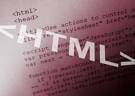0
Thanks

A few words of thanks would be greatly appreciated.

# HTML - For loop with if and else## Issue

I must create a pop-up that will be triggered upon answering a question.
E.g 3+1 =
My problem comes when the user is anything other than the number 4, the question will be asked again (in a loop), until the right answer is given.

```<script type="text/javascript">
nom = window.prompt("nom ?");
</script>

<script type="text/javascript">

nb = window.prompt("3+1 ?");

if (nb==4) {
}
else { nb!=4
nb = window.prompt("3+1 ?");
} ```

## Solution

Do not use a for loop, but a while.

Replaces your second script with this:

```<script type="text/javascript">
nb = 0;

while (nb != 4){
nb = window.prompt("3+1 ?");
}
if (nb == 4){
}
</script>
```
0
Thanks

A few words of thanks would be greatly appreciated.